# KSEEB Solutions for Class 7 Maths Chapter 3 Data Handling Ex 3.1

Students can Download Class 7 Maths Chapter 3 Data Handling Ex 3.1 Questions and Answers, Notes Pdf, KSEEB Solutions for Class 7 Maths helps you to revise the complete Karnataka State Board Syllabus and to clear all their doubts, score well in final exams.

## Karnataka State Syllabus Class 7 Maths Chapter 3 Data Handling Ex 3.1

Question 1.
Organise the following marks ion a class assessment, in a tabular form.
4, 6, 7, 5, 3, 5, 4, 5, 2, 6, 2, 5, 1, 9, 6, 5, 8, 4, 6, 7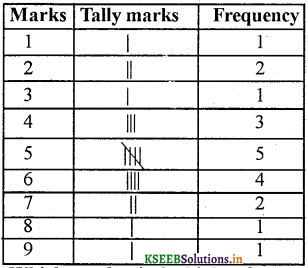i) Which number is the highest?
Solution:
Highest number is 9

ii) Which number is the lowest?
Solution:
Lowest number is 1iii) What is the range of the data?
Solution:
Range of the given data = Highest number – Lowest number = 9 – 1 = 8

iv) Find the arithmetic mean.
Solution:
Arithmetic Mean of marks in the class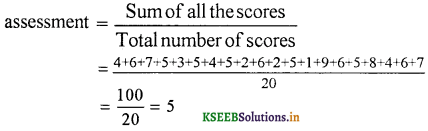Question 2.
Find the mean of the first five whole numbers.
Solution:
The first whole numbers are 0, 1, 2, 3, 4
Arithemetic mean of five whole numbers =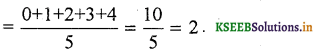Question 3.
A cricketer scores the following runs in eight innings :
58, 76, 40, 35, 46, 45, 0, 100. Find the mean score.
Solution:
The scores of 8 innings = 58, 76, 40, 35, 46, 45, 0, 100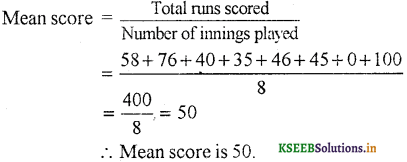Question 4.
Following table shows the points of each player scored in four games :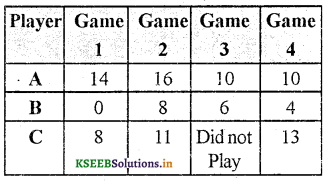i) Find the mean to determine as average number of points scored per game.
Solution:
A’s average number of points scored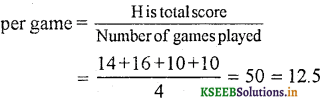ii) To find the mean number of points per game for C, would you divide the total points by 3 or 4? Why?
Solution:
To find the mean number of points per game for C, we shall divide the total points by 3 only because he did not play the 3rd game.

iii) B played in all the four games. How would you find the mean?
Solution:
‘B’ played all the four games. So we findiv) A is the best performerQuestion 5.
The marks (out of 100) obtained by a group of students in a science test are 85, 76, 90, 85, 39, 48, 56, 95, 81 and 75. Find the:
Solution:
Marks obtained by a group (in ascending order) = 39, 48, 56, 75, 76, 81, 85, 85, 90, 95
i) Highest and lowest marks obtained by the students.
Solution:
Highest and the lowest marks obtained by the student 95 and 39 respectively

ii) Range of the marks obtained.
Range = Highest score – Lowest score
= 95 -39 = 56

iii) Mean marks obtained by the group.Question 6.
The enrollment in a school for six consecutive years was as follows :
1555, 1670, 1750, 2013, 2540, 2820. Find the mean enrollment of the school for this period.
Solution:
Mean enrollment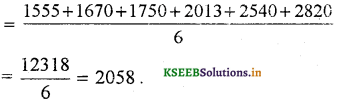Question 7.
The rainfall (in mm) in a city on 7 days of a certain week was recorded as follows: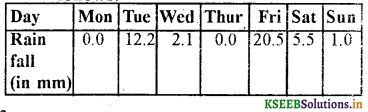i) Find the range of rainfall in the above data.
Solution:
Range of the rainfall = Highest Rainfall – Lowest Rainfall
= 20.5 – 0.0 = 20.5

ii) Find the mean rainfall for the week
Solution: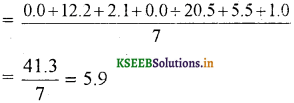iii) On how many days was the rainfall less than the mean rainfall.
Solution:
No. of days of rainfall which was less than Mean rainfall } = 5 daysQuestion 8.
The heights of 10 girls were measured in cms and the results are as follows: 135, 150, 139, 128, 151, 132, 146, 149, 143, 141.
Solution:
The height of girls are arranged in ascending order} = 128, 132, 135, 139, 141, 143, 146, 149, 150, 151.

i) What is the height of the tallest girl?
Solution:
The height of the tallest girl is 151 cms

ii) What is the height of the shortest girl?
Solution:
The height of the shortest girl is 128cms

iii) What is the range of the data?
Solution:
The range of the data = Highest height – lowest height
= 151 – 128
= 23

iv) What is the mean height of the girls?
Solution:
Mean height of the girlsv) How many girls have heights more than the mean height?
Solution:
No. of girls who were more than mean height = 5